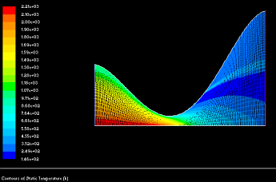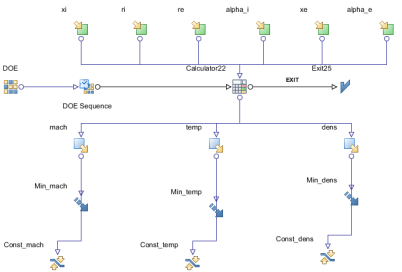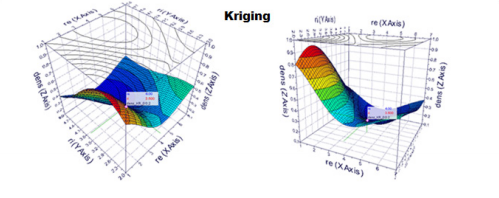Design Optimization of Nozzle Shapes for Maximum Uniformity of Exit Flow

Author(s):
Karla Keldani Quintão (Florida International University)

The objective of this study is to identify the optimal designs of converging-diverging supersonic and hypersonic nozzles that perform at maximum uniformity of thermodynamic and flow-field properties with respect to their average values at the nozzle exit. The converging – diverging nozzles are used to accelerate the fluid to supersonic speeds past the throat of such a nozzle.Exit flow from a converging-diverging nozzle often has strong gradients of pressure, temperature, density, and speed in radial and axial direction. These non-uniformities at the nozzle exit are the result of the non-uniformities in the flow-field entering the nozzle. Since this is a multi-objective design optimization problem, the design variables used are parameters defining the shape of the nozzle. This work presents how variation of such parameters can influence the nozzle exit flow non-uniformities. ANSYS GAMBIT was used for mesh generation and ANSYS FLUENT for flow analysis. The analyses were made for two turbulence models. A non-uniform temperature distribution profile at the inlet was used to simulate different conditions. ANSYS FLUENT was used to simulate the compressible, viscous gas flow-field in forty nozzle shapes, including the heat transfer analysis. The results of two turbulence models, k-ε and k-ω, were computed and compared. To significantly accelerate the entire design optimization process, response surfaces (meta-models) were generated using the Kriging and RBF (Radial Basis Function) algorithms in order to perform the virtual optimization. The optimization was performed withmodeFRONTIER software package using Kriging and Radial Basis Functions (RBF) response surfaces. The optimization was run using MOGA-II algorithm. The Pareto solutions from the optimization were validated by randomly choosing one of the virtual designs generated and using its 53 values for the design variables in ANSYS FLUENT and computing the solutions for density, Mach number and temperature standard deviations. In order to compare both solutions, the calculated errors were rather large. By running the optimization of the same problem, but using another optimization algorithm (MOPSO) instead of MOGA II, it could be observed that although the errors of the response surfaces were large, the values of Mach number and temperature standard deviations calculated in ANSYS FLUENT were smaller than those when optimization was done by using genetic algorithm. This can represent a better capacity of particle swarm to find the global minimal.